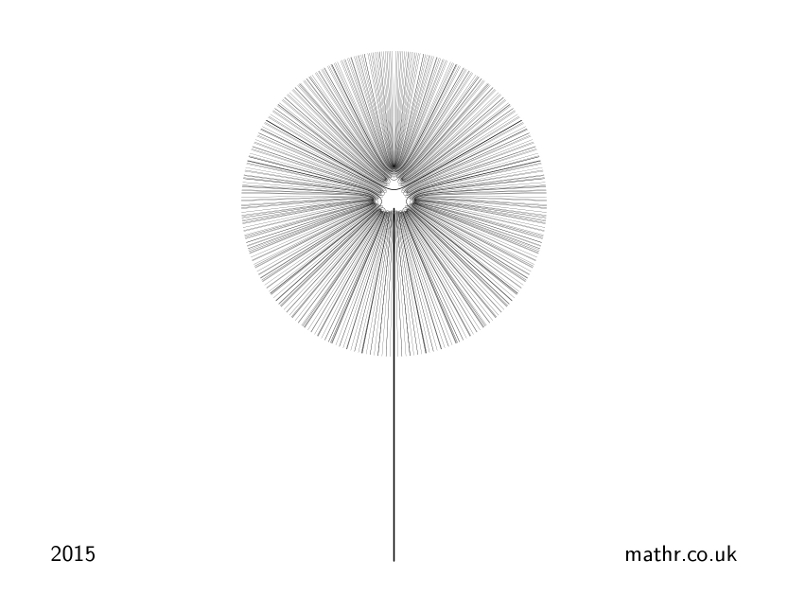# mathr / blog / #

## Calendar 2015 - DandelionA shadow of the Mandelbrot set formed by periodic external rays. See the start of my previous post for some context as to what periodic external rays are. Periodic external angles can be expressed as $$\frac{m}{2^p-1}$$ though this fraction may not be in lowest terms. Not all $$m$$ give a period $$p$$ external angle, for some the true period is lower, so this needs to be checked to avoid drawing rays more than once.

The Dandelion is formed by drawing all the periodic rays up to a maximum period of 8, clipped to a circle centered on the origin (apart from the period 1 ray which extends to form the stalk). Lower periods have thicker lines. Periodic rays land in pairs at the roots of hyperbolic components, and never enter the interior of the Mandelbrot set, so the shape of the Mandelbrot set is visible as a shadow in the center of the Dandelion. In the limit with no maximum period, the Dandelion would be a solid black circle with the components of the Mandelbrot set visible in white.

Here's a brief extract from the source code, lightly modified to remove some less important details:

for (int period = 2; period <= maxperiod; ++period) {
cairo_set_line_width(cr, weight / period);
int denominator = (1 << period) - 1;
for (int numerator = 1; numerator < denominator; ++numerator) {
mpq_set_si(angle, numerator, denominator);
mpq_canonicalize(angle);
// check that the period is correct
struct mandelbrot_binary_angle *b = mandelbrot_binary_angle_from_rational(angle);
int bperiod = mandelbrot_binary_angle_period(b);
mandelbrot_binary_angle_delete(b);
if (period != bperiod) { continue; }
// trace the ray
struct mandelbrot_external_ray_in_native *ray = mandelbrot_external_ray_in_native_new(angle);
complex double endpoint = to_screen(mandelbrot_external_ray_in_native_get(ray));
cairo_move_to(cr, creal(endpoint), cimag(endpoint));
for (int i = 0; i < raysteps; ++i) {
mandelbrot_external_ray_in_native_step(ray);
endpoint = to_screen(mandelbrot_external_ray_in_native_get(ray));
cairo_line_to(cr, creal(endpoint), cimag(endpoint));
}
cairo_stroke(cr);
mandelbrot_external_ray_in_native_delete(ray);
}
}

The full source code dandelion.c uses the library in my book project repository book project repository. Unfortunately it seems that that repository has grown to over 60MB, so I'll try to find some time soon to filter it into two repositories, one of which will be much smaller (containing useful re-usable code).

Note: I lost the original source code in the depths of my drives, this is a recreation with parameters tuned to match the original image.